# ECE5725 Project: Parking Lot

Really Awesome
A Project By Xinyu Wu & Shiqi Li.

## Introduction

Today, vehicles are widely used and parking a car becomes an inevitable problem. One situation people may encounter is that a car needs to park in a designated parking spot, for example, a private parking spot marked with a license plate number, and drivers can easily forget where their parking slots are. This project aims to design a robot car that can recognize license plate numbers in a parking lot and back itself into the corresponding location with its license plate number. In our parking lot, license plate numbers are composed of letters and numbers which simulate the real-world situation. In this project, the robot was placed at a starting line to detect the right parking spot. We used the RPi to control the movement of the robot. The car was controlled to start finding the parking slot by pressing the button GPIO 17 on the piTFT manually. We also implemented the function that the robot can direct itself into the parking slot autonomously. We designed a program on the RPi so that the robot could memorize a randomly generated input license plate number from a license plate list which contained three license plate numbers and it compared the generated license plate number with detected numbers. A Picamera attached to the RPi on the right side of the car was used to detect license plate numbers in the parking lot. We developed an image processing algorithm to process the image captured and to recognize and compare the specific license plate number. The captured real-time picture could be projected on the piTFT for the convenience of observation. If it detects the right parking spot, the robot will turn right at an angle of 90 degrees and park itself into the spot.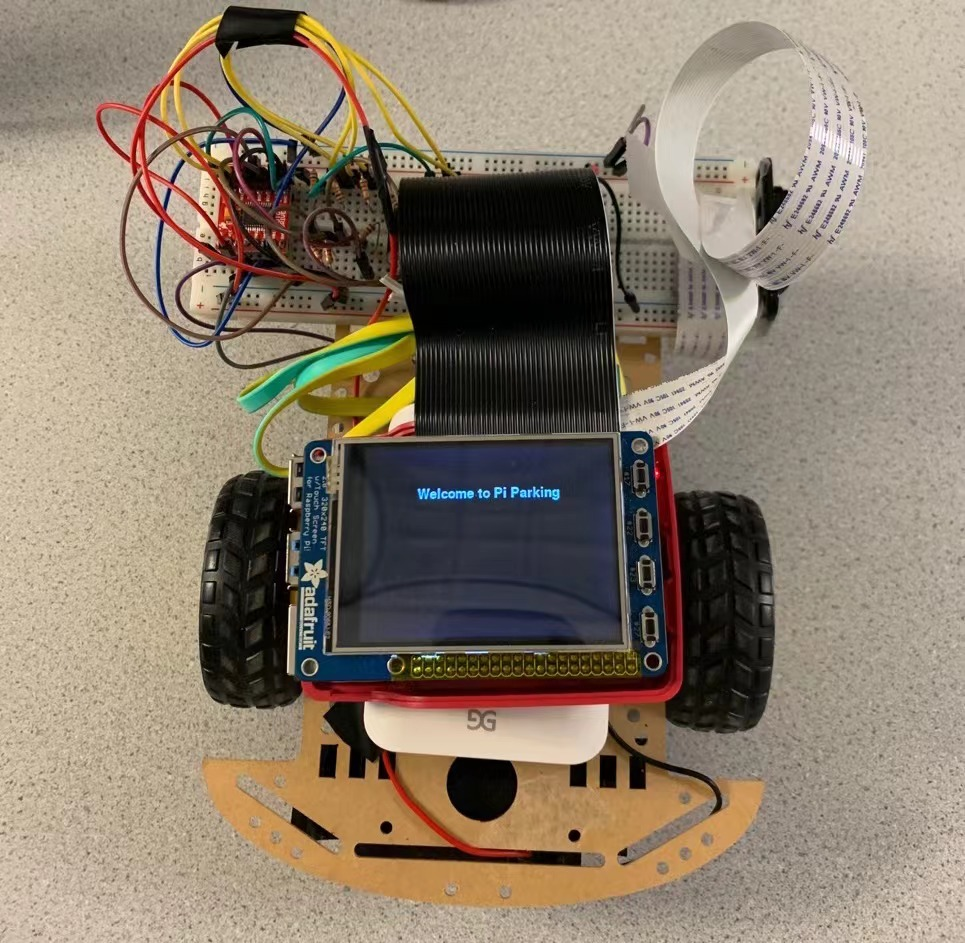## Project Objective:

• The car is autonomous with a physical start button and a physical quit button.
• The car’s license plate number is randomly generated by the program.
• The Pi camera attached to the robot can detect the license plate number at each parking spot and compared with the input license plate number.
• The robot can move along the road and park itself in front of the right license plate with implemented control program.
• The captured real-time images and both the target and the detected license plate numbers can be projected on the piTFT for the convenience of observation.

## Design and Testing

Assemble the robot car referring to Lab3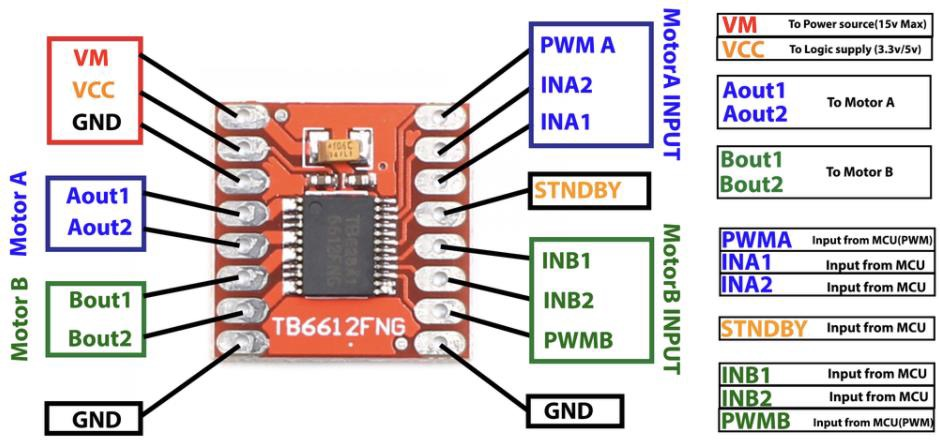Figure 1. Motor Controller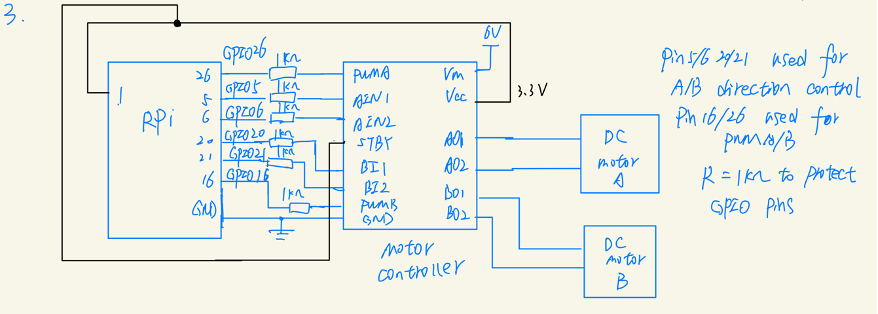Figure 2. Circuit Schematic

The first figure shows the connections for the motor controller. The second diagram is a circuit connection diagram and from Lab3. We referred to the diagram and reassembled our robot car. To drive the dc motors, we needed a Sparkfun TB6612FNG dual-channel motor controller which was connected to the RPi and dc motors. Here, RPi was powered with 5V, the motor controller was powered with 3.3V by RPi, and the dc motor was powered with 6V. 1K resistors were used in the circuit to protect GPIO pins.

Configure the Pi camera

We aimed to install OpenCV on the RPi Linux system based on the Lab4’s kernel system which was 5.10.25 Preempt RT version. We followed the process given on Canvas and used the command `sudo apt-get install libopencv-dev python-opencv` to install OpenCV. Then we tried to install the Picamera module. The camera module ribbon cable was inserted into the camera port on the RPi. One thing worth noting was that the connectors at the bottom of the ribbon cable were facing the contacts in the port. Our camera ribbon cable was broken down due to some contact problem and we replaced it by a new one during the testing process at the later stage of our project. After inserting the camera module, we configured the RPi by enabling the camera module in Interfaces in the RPI configuration window. When rebooting completed, we tested our camera module by using the command: `raspistill -o Desktop/image.jpg ` The captured file was saved as “image.jpg”. We tested the camera which turned out to be normally functioning by using the above command and checking the saved image.

Set up the environment

During the installation of OpenCV, we encountered a problem. The original version of python on the kernel system we used was 2.0+ while one tool needed to recognize characters required python 3.0+. Thus, we updated the version of Python and when we tried to run “sudo apt-get upgrade” to install available upgrades of all packages currently installed on the system, the upgrade process kept running and we mistakenly killed the process, which resulted in the system breaking down. Fortunately, we have backed up the system, and we simply recover the system from the previous backup file.
To update the Python version, we issued the command to install python3.6 version: Sudo apt-get install python3.6 Next, we followed the instructions on Canvas to install OpenCV on Python 3.6.

``` pip3 install opencv-contrib-python==3.4.6.27 -i sudo apt-get update #setup of dependencies sudo apt-get install libhdf5-dev sudo apt-get install libatlas-base-dev sudo apt-get install libjasper-dev sudo apt-get install libqt4-test sudo apt-get install libqtgui4 sudo apt-get update ```

When using the command `pip3 install opencv-contrib-python==3.4.6.27 -i` to install opencv, the installed package “cv2” can only be run without the “sudo” command. To fix this problem, we added “sudo” to install opencv and “cv2” can be imported when using “sudo python3”.

In addition, we kept receiving error messages concerning the unmet dependencies even though we added “sudo” in each command. After trying abundant methods, we fixed the problem by adding “-t buster” to the end of a command when using sudo apt-get install [package]. For example, when issuing the command
`sudo apt-get update #setup of dependencies`

We should use
`sudo apt-get install libhdf5-dev -t buster`

In this way, all the required dependencies could be resolved automatically rather than being manually downloaded one by one.

Another problem we encountered was the version of “pip” which used to be 2.7 and was incompatible with the pytesseract package. We solved this problem by using
`python3 -m pip install pytesseract`

Process detected images

We developed an image processing algorithm to capture and process images. Our license plate numbers are composed of both letters and numbers. Thus, we made three license plates and printed them out.

At first, we tried to process the original images by issuing a command involving pytesseract. The results derived turned out to be highly inaccurate. Sometimes there were no results after using pytesseract or there were unrecognized characters in the results. Therefore, we decided to develop an image preprocessing algorithm.

Capture images  by Pi Camera

First, we used Picamera to capture a picture and save as image.jpg. We imported picamera library to get the picture. Images can be taken by using:

``` from picamera import PiCamera from time import sleep camera = PiCamera() camera.start_preview() time.sleep(0.2) camera.capture('image.jpg') camera.stop_preview() ```

Note If your preview is upside-down, you can rotate it by 180 degrees with the following code:

`camera.rotation = 180`

The original picture is shown as follows: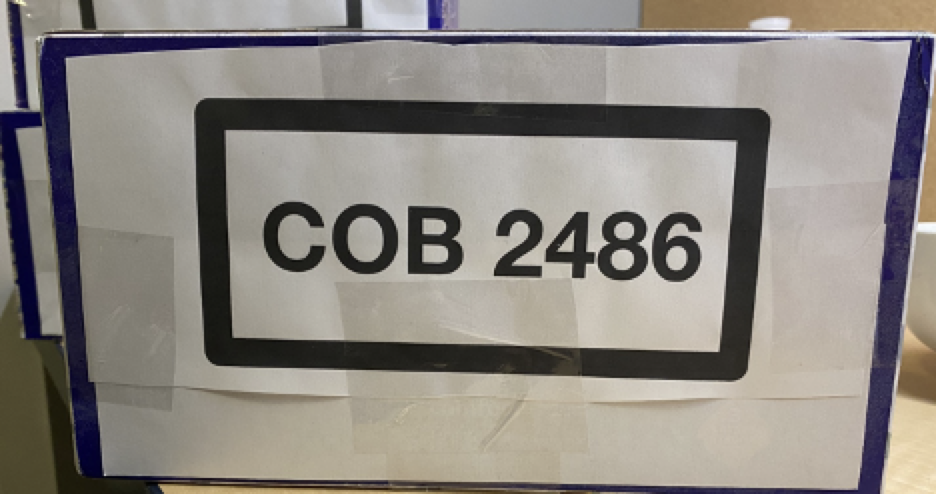Figure 3. Original image
Perform Image preprocessing

Then we process the image for further character recognition including characters localization, background deletion, noise reduction, and characters enhancement.
First, we preprocessed the initial image and localized the characters by splitting the black rectangular area and removing the background area. This improves the accuracy of character recognition. The processed image is saved as "preprocess.jpg".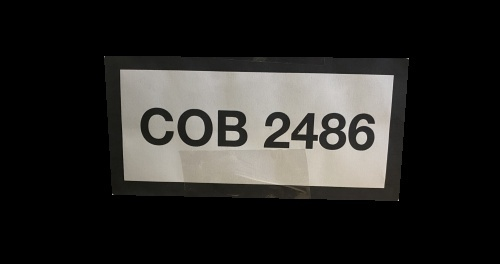Figure 4. Preprocessed image to split the black rectangular region

Then read the preprocess.img with the following code:

`img = cv2.imread("preprocess.jpg")`

Convert the image to a grayscaled image with the following code:

`img_gray = cv2.cvtColor(img, cv2.COLOR_BGR2GRAY)`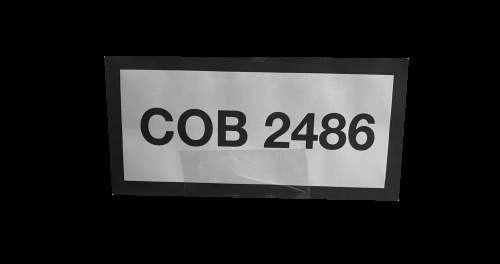Figure 5. Grayscaled image

Use Morphological Transformations to maximize contrast with the following code:

```structuringElement = cv2.getStructuringElement(cv2.MORPH_RECT, (3, 3)) imgTopHat = cv2.morphologyEx(img_gray, cv2.MORPH_TOPHAT, structuringElement) imgBlackHat = cv2.morphologyEx(img_gray, cv2.MORPH_BLACKHAT, structuringElement)```

Use findContours function to find the contours of characters:

```binary, contours, hierarchy = cv2.findContours( img_thresh, cv2.RETR_LIST, cv2.CHAIN_APPROX_SIMPLE )```

Note: In OpenCV 3.x this function needs to return 3 values which is different from the OpenCV 2.x version.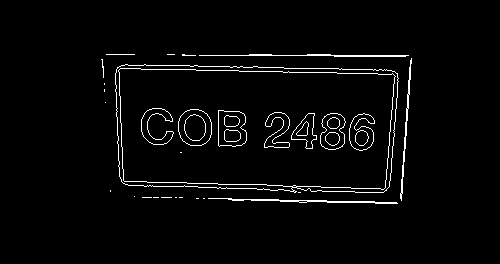Figure 6. Image processed with findContours

Draw contours using drawContours function:

```# black temp pic temp_result = np.zeros((height, width, channel), dtype=np.uint8) # draw contours cv2.drawContours(temp_result, contours=contours, contourIdx=-1, color=(255, 255, 255))```

Visualize possible contours of characters: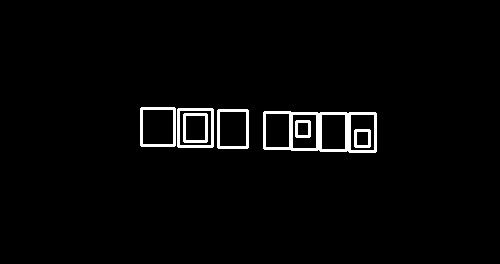Figure 7. Image processed with drawContours

Splitting character areas using getRectSubPix( ):

```img_cropped = cv2.getRectSubPix( img_rotated, patchSize=(int(plate_width), int(plate_height)), center=(int(plate_cx), int(plate_cy)) ) ```

Crop characters to derive the following picture: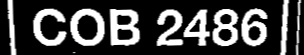Figure 8. Cropped characters

Finally, make the characters bigger with copyMakeBorder() function to generate the picture result and save it as "result.jpg":

```img_result = cv2.copyMakeBorder(img_result, top=10, bottom=10, left=10, right=10, borderType=cv2.BORDER_CONSTANT, value=(0, 0, 0))```

Here is the generated final result: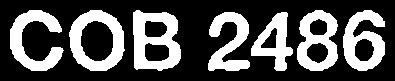Figure 9. Final result

Use tesseract engine to recognize the characters by running:

`tesseract result.jpg file -l eng -psm 7`

The detection results are saved in the "file.txt".
We got the detection results by reading this file with the following codes:

```# detect the license plate from the streaming picture f = open("file.txt", "r") text = f.readline() text = text.replace("\n", "") f.close()```

Then by comparing the results and target license plate, we can know whether the robot should move forward or stop here.

Design the car control algorithms

We utilized PWM to control the left and right motors. A PWM instance can be created using `p = GPIO.PWM(26, frequency)`   and can be started using `p.start(dutycycle)`. To change the duty cycle of a pulse width modulation signal, we use `pa.ChangeDutyCycle(dc)`. Since our robot car moves slowly in order to capture images and detect during the movement, the dutycycle was set to be about 70. To ensure the car moves in a straight line and to avoid inconsistency in speeds of two motors, we adjusted the two duty cycles to be different. When the car needs to turn right to park in front of the right license plate, the left motor moves forward while the right motor moves backward.

## Flow Chart

Here are three flow charts describing image processing and car control algorithms.

This flow chart describes the whole picture of the software control algorithm including both image processing and car control.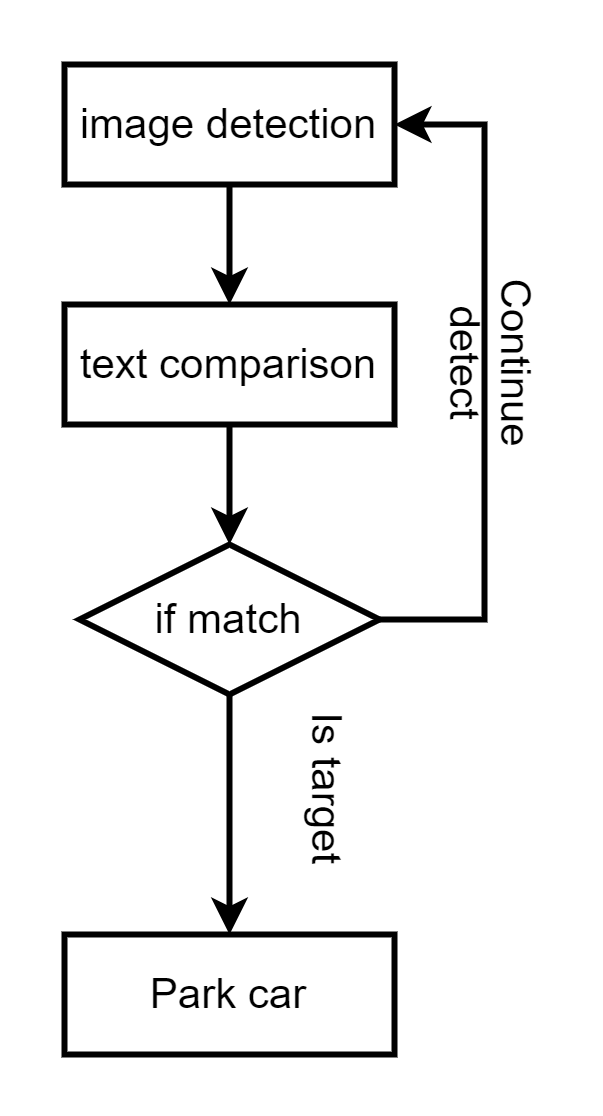The following flow chart describes the image processing algorithm.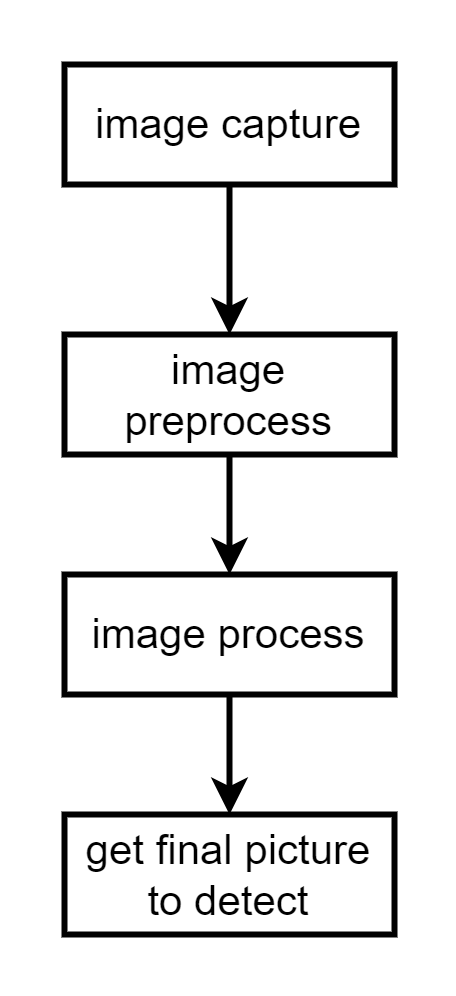The following flow chart describes the car control algorithm.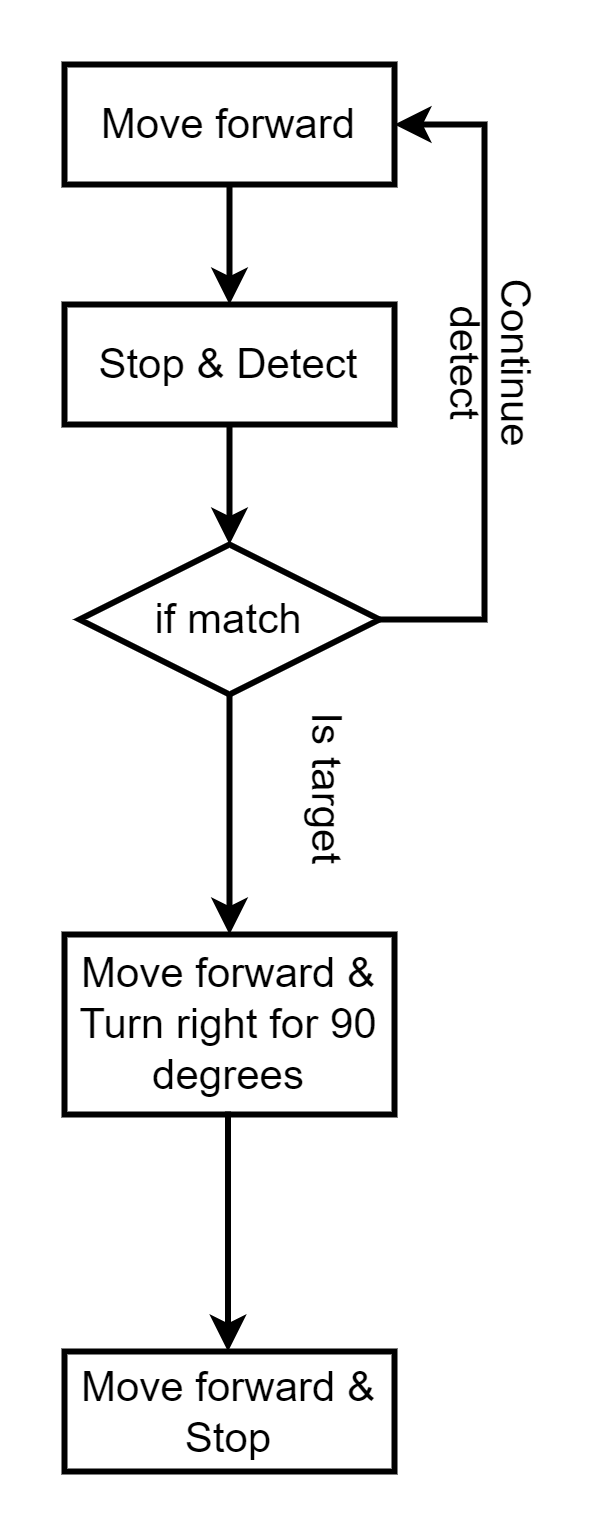## Result

We eventually reached the goal of our project. In the software part, our robot car could successfully capture images using the Picamera. The car would take pictures each time it drives forward for a distance and stops. When a picture is taken, the program starts to process the taken images. A preprocessing algorithm is applied before the RPi begins to recognize the characters in pictures. After preprocessing images, characters will appear to be more clear and easy to be recognized by using pytesseract. The results recognized will be output to a file and can be read by the program. The recognized license plate number will also be printed on the piTFT screen, together with the original captured image and the target license plate number. The following three pictures show three kinds of situations where three license plates are detected and shown on the piTFT. The robot compares the target license plate number with the recognized characters and sees whether there is a match. If the target matches the derived texts, the robot will direct itself into the location in front of the corresponding license plate number. The program ends when the right license plate is detected, even though there are still license plates not being detected. Otherwise, the robot car moves forward and continues detecting until the right license plate is found.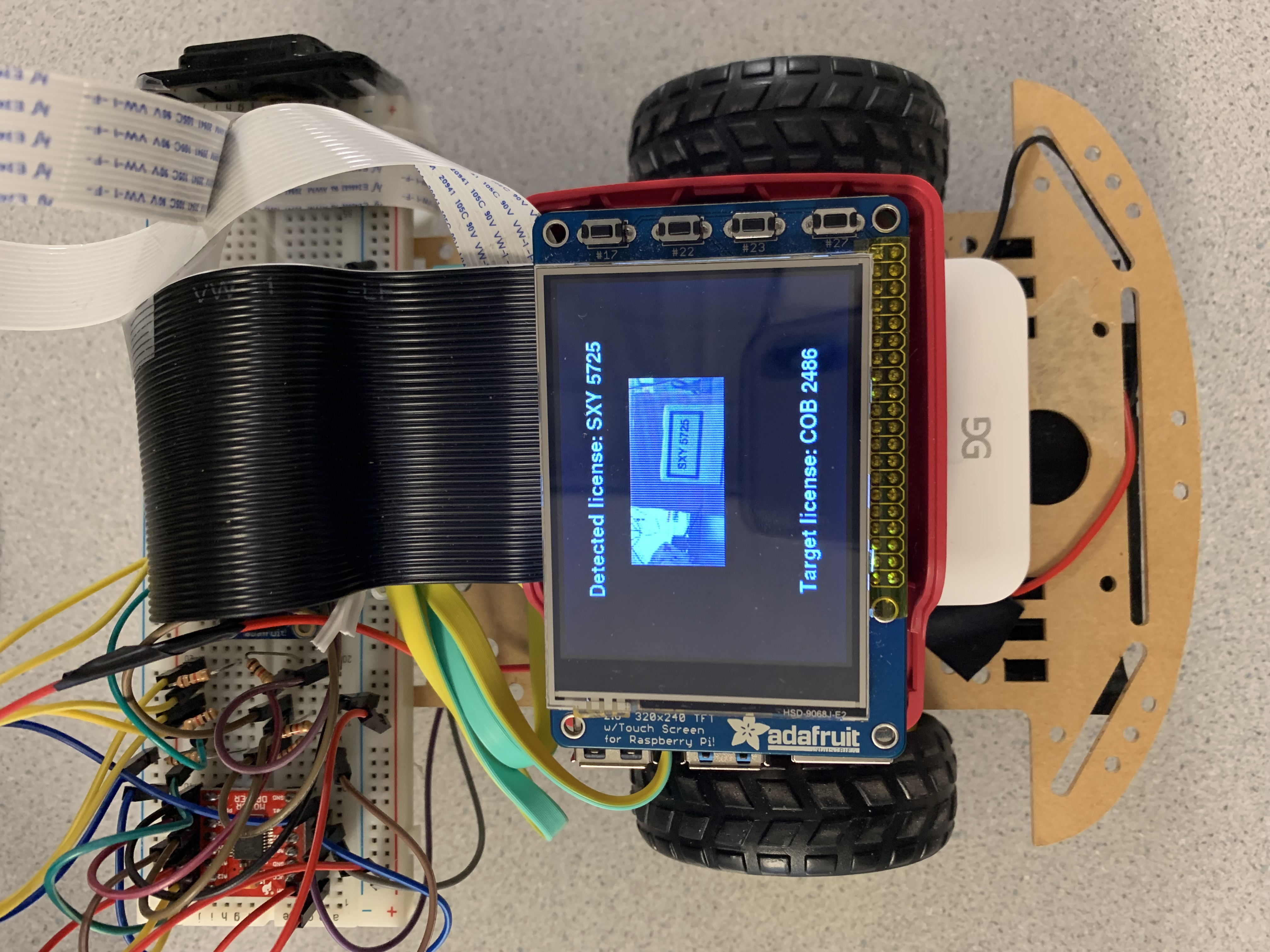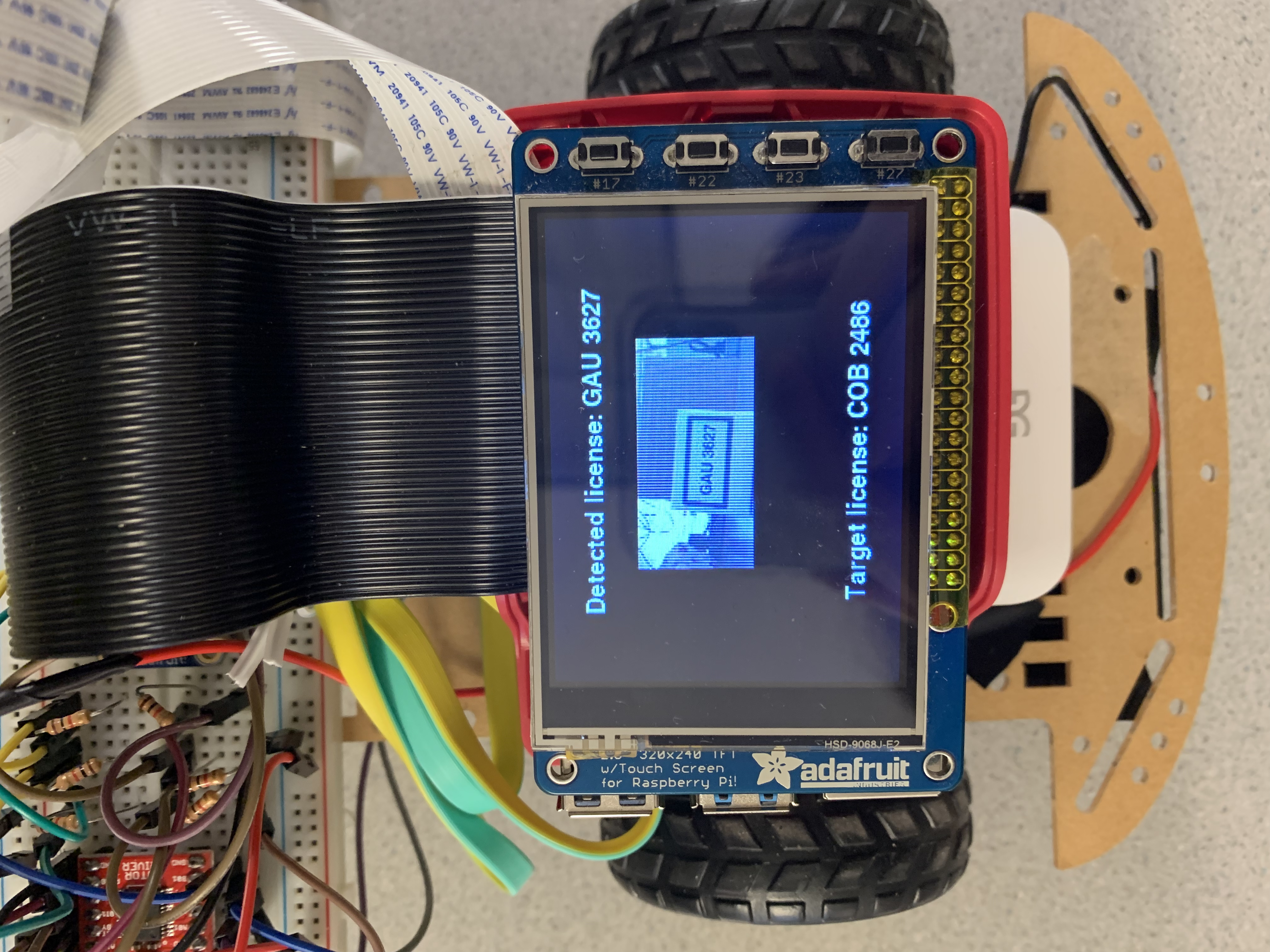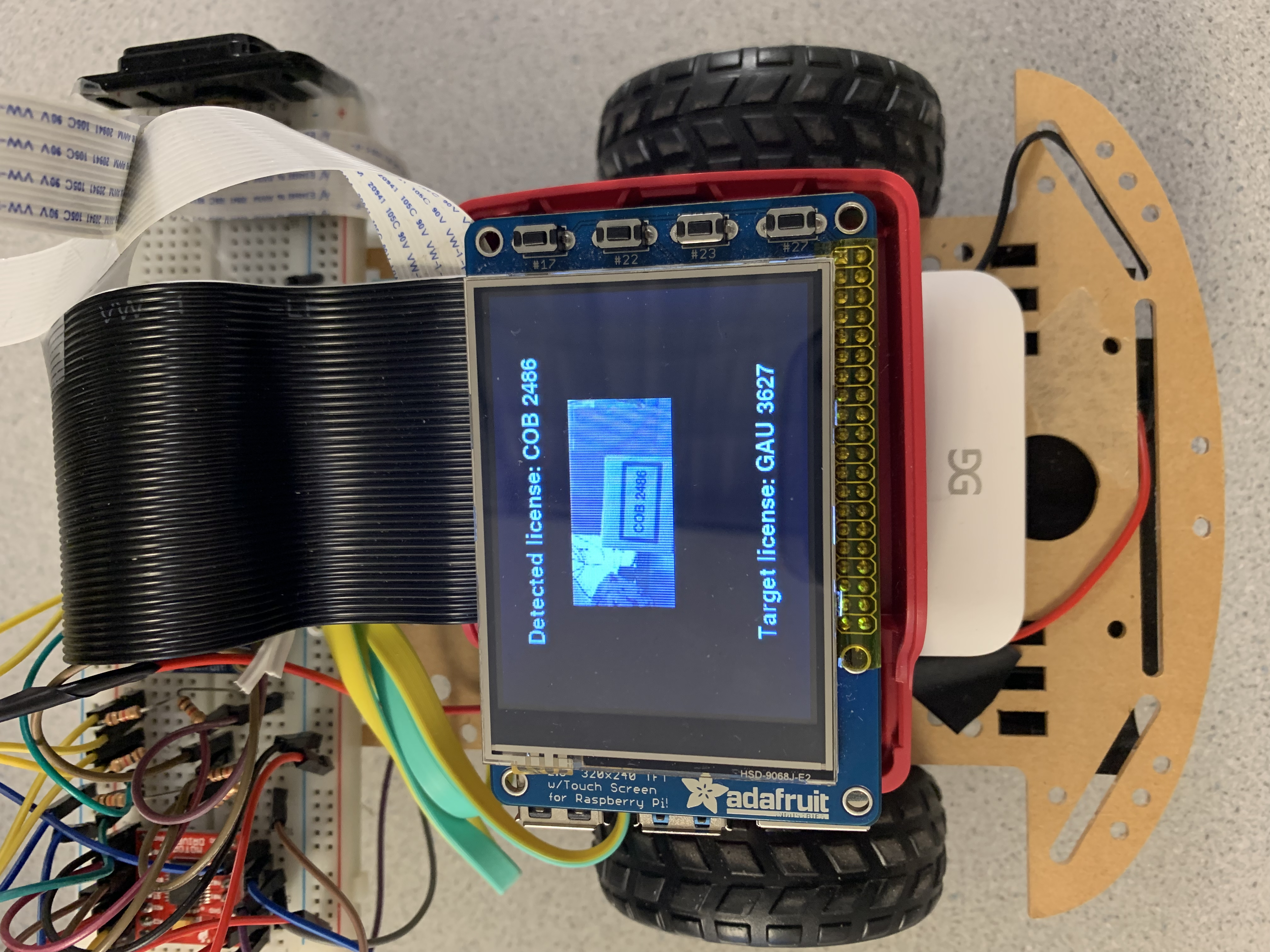Detected license plate: SXY 5725, GAU 3627, COB 2486

In the hardware part, the robot car is initialized with the text “Welcome to Pi Parking '' shown on the piTFT screen. The program starts with the physical button being pressed, which in our case is GPIO 17 on the piTFT. Then a target license plate is generated from a list of three picked license plates by using choice() function from “random” library. The car slowly moves straight forward and stops to capture an image. If the right license plate is detected, the car turns right and parks itself in front of the corresponding license plate. If not, the car moves forward and repeats to detect until the right position is found.

## Conclusion and Future Work

We designed and tested a parking lot with an autonomous robot car. We successfully reached our goals by developing image processing and car control algorithms in software using OpenCV and PWM signals. We also set up a parking lot and constructed our own robot car. To detect images using the camera, we installed the camera on the right side of the car so that the car could detect during its movement. For the future work, we could consider develop a closed-loop control algorithm to give the car some feedbacks regarding its movement so that the car would move forward in a straight line more easily without adjusting the parameters in our code. Another possible future work would be to develop a parking algorithm with some sensors so that the car would park in a specific slot more accurately.

## Work Distribution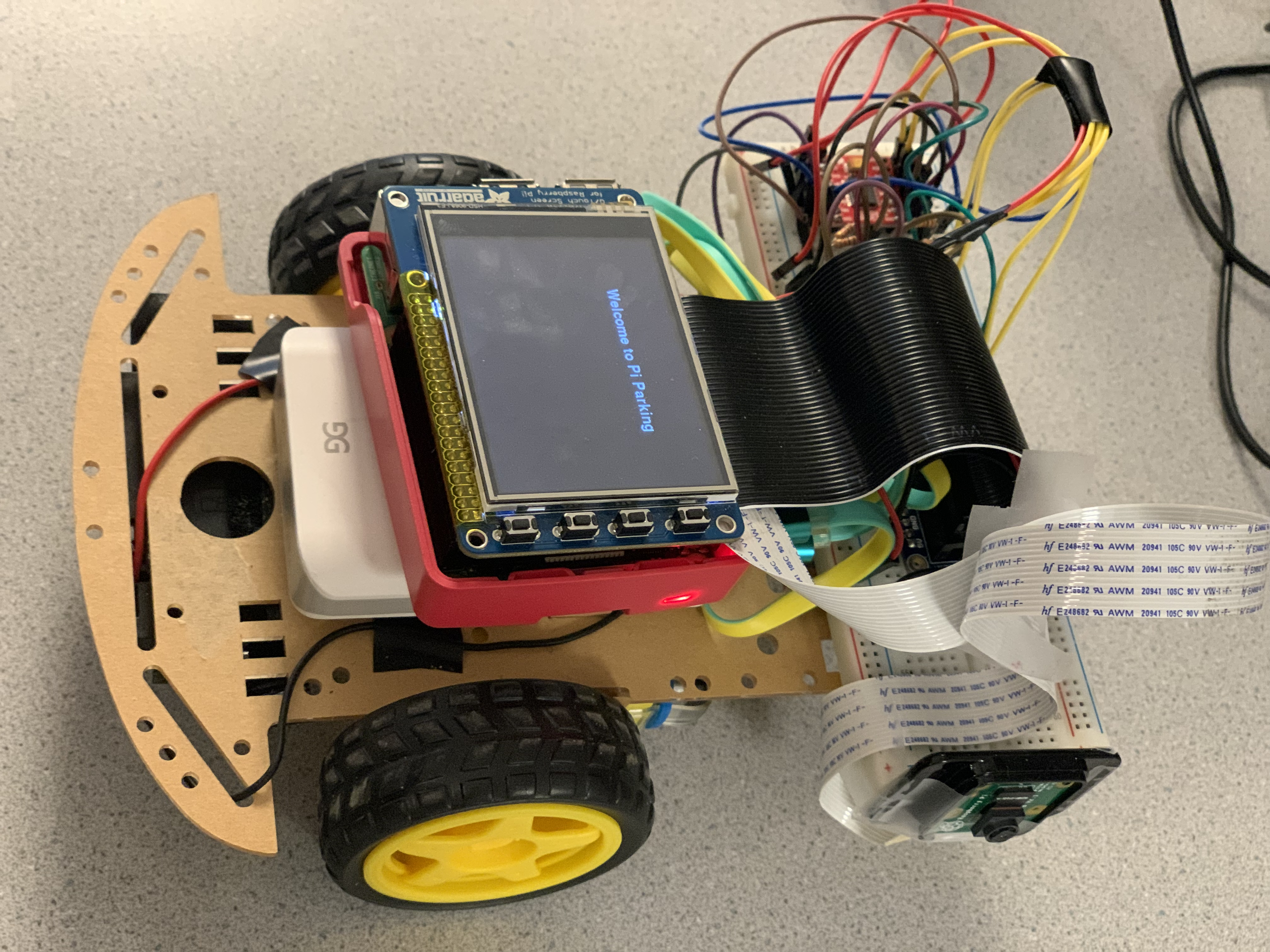#### Project group picture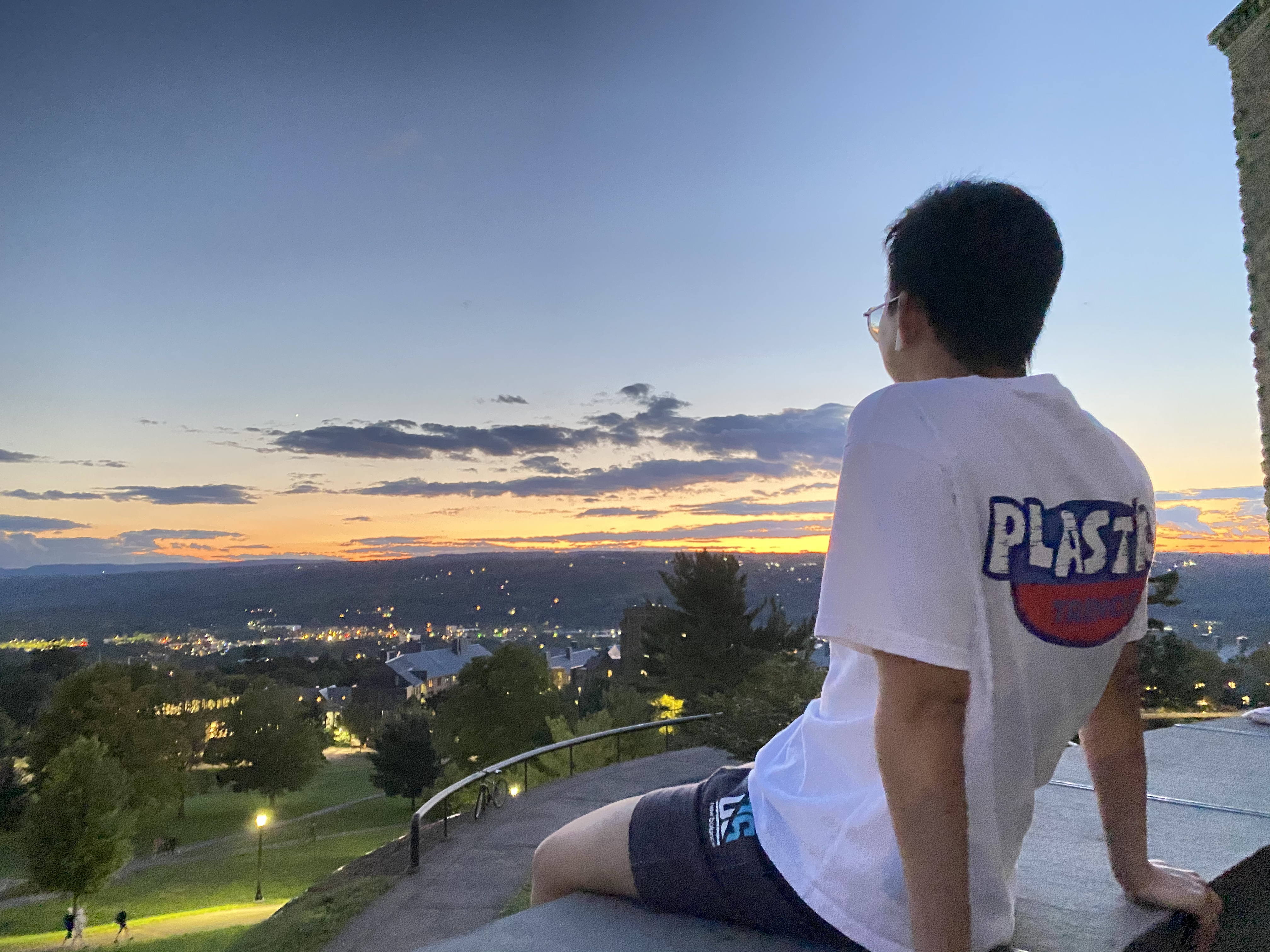### Xinyu Wu

xw586@cornell.edu

Designed the image processing algorithm and tested the overall system.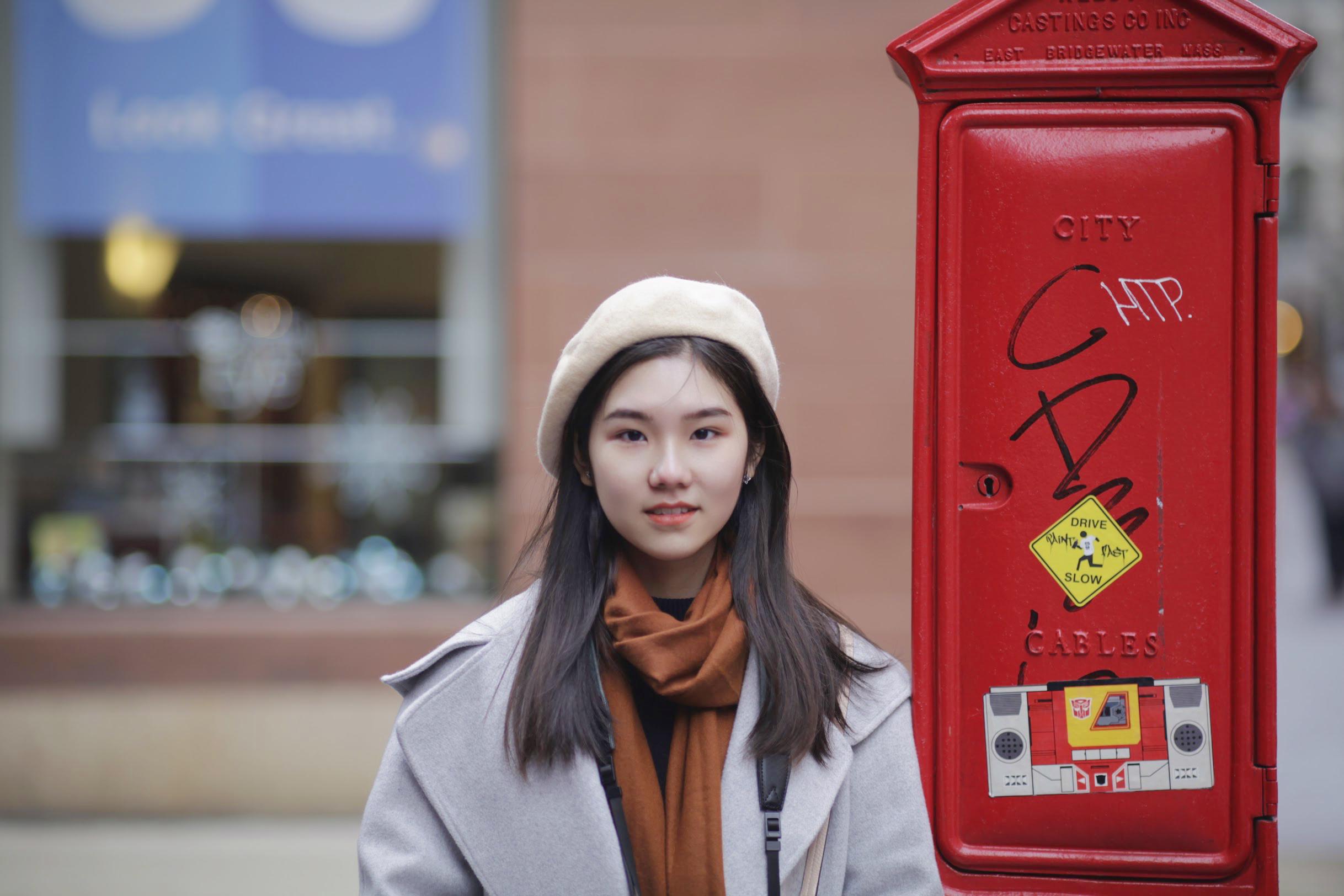### Shiqi Li

sl2454@cornell.edu

Designed the display and car control algorithm and tested the overall system.

## Parts List

• Raspberry Pi \$35.00
• Motor Driver TB6612FNG \$4.95
• Wheel robot platform \$24.99
• Raspberry Pi Camera V2 \$25.00

### Total: \$0 (Provided from lab)

```# Opencv
import cv2
import numpy as np
from picamera import PiCamera
# import pytesseract
from random import choice
# car control
import time
import RPi.GPIO as GPIO
import sys, pygame
from pygame.locals import *
import os
from PIL import Image

# run cmd
import subprocess

# ======================= GPIO pygame Initialize ===========================
os.putenv('SDL_VIDEODRIVER', 'fbcon')  # Display on piTFT
os.putenv('SDL_FBDEV', '/dev/fb0')  #
os.putenv('SDL_MOUSEDRV', 'TSLIB')  # Track mouse clicks on piTFT
os.putenv('SDL_MOUSEDEV', '/dev/input/touchscreen')

GPIO.setmode(GPIO.BCM)  # Set for broadcom numbering not board numbers...
# PWM control
GPIO.setup(26, GPIO.OUT)  # set GPIO 26 as PWMA
GPIO.setup(16, GPIO.OUT)  # set GPIO 16 as PWMB
# direction control
GPIO.setup(5, GPIO.OUT)  # set GPIO 5 as AIN1
GPIO.setup(6, GPIO.OUT)  # set GPIO 6 as AIN2
GPIO.setup(20, GPIO.OUT)  # set GPIO 20 as BIN1
GPIO.setup(21, GPIO.OUT)  # set GPIO 20 as BIN1
# button control
GPIO.setup(17, GPIO.IN, pull_up_down=GPIO.PUD_UP)
GPIO.setup(22, GPIO.IN, pull_up_down=GPIO.PUD_UP)
GPIO.setup(23, GPIO.IN, pull_up_down=GPIO.PUD_UP)
GPIO.setup(27, GPIO.IN, pull_up_down=GPIO.PUD_UP)

# colors
WHITE = (255, 255, 255)
RED = (255, 0, 0)
GREEN = (0, 255, 0)
BLACK = (0, 0, 0)
size = width, height = 320, 240
# pygame initialize
pygame.init()
pygame.mouse.set_visible(False)
screen = pygame.display.set_mode((320, 240))  # screen layout
my_font = pygame.font.Font(None, 25)

# ============================== Car Control ==================================
fa = 50
fb = 50
dca = 0
dcb = 0
# To create a PWM instance:
pa = GPIO.PWM(26, fa)
pb = GPIO.PWM(16, fb)

# To start PWM:
pa.start(dca)  # where dc is the duty cycle (0.0 <= dc <= 100.0)
pb.start(dcb)

def control_servo(serv_num, direction, dc):
# Motor A
if serv_num == "left":
# CW
if direction == 'cw':
GPIO.output(5, GPIO.HIGH)
GPIO.output(6, GPIO.LOW)
pa.ChangeDutyCycle(dc)
if direction == 'ccw':
GPIO.output(5, GPIO.LOW)
GPIO.output(6, GPIO.HIGH)
pa.ChangeDutyCycle(100)
if direction == 'stop':
pa.ChangeDutyCycle(0)

if serv_num == "right":
if direction == 'cw':
GPIO.output(20, GPIO.HIGH)
GPIO.output(21, GPIO.LOW)
pb.ChangeDutyCycle(dc)
if direction == 'ccw':
GPIO.output(20, GPIO.LOW)
GPIO.output(21, GPIO.HIGH)
pb.ChangeDutyCycle(dc)
if direction == 'stop':
pb.ChangeDutyCycle(0)

# detecting if text equals to the target texts
match = False

def car_control(iftext):
'''
iftext: detecting if text equals to target
if equals car turn around into the position
else moving forward
'''
if iftext:
# if match target license, turn right
# go straight
control_servo("left", 'cw', 65)
control_servo("right", 'ccw', 73)
time.sleep(1)
# turn right
control_servo("left", 'cw', 90)
control_servo("right", 'cw', 96)
time.sleep(0.75)
# move forward
control_servo("left", 'cw', 65)
control_servo("right", 'ccw', 73)
time.sleep(1.7)
# continue to turn right
#control_servo("left", 'cw', 90)
#control_servo("right", 'cw', 96)
#time.sleep(0.15)
control_servo("left", 'stop', 0)
control_servo("right", 'stop', 0)
# else:
#    control_servo("left", 'stop')
#    control_servo("right", 'stop')

def image_resize(filename):
baselen = 160
image = Image.open(filename)
w, h = image.size
if w <= baselen and h <= baselen:
print(filename, 'is in proper size.')
return
if (1.0 * w / baselen) > (1.0 * h / baselen):
scale = 1.0 * w / baselen
new_im = image.resize((int(w / scale), int(h / scale)), Image.ANTIALIAS)

else:
scale = 1.0 * h / baselen
new_im = image.resize((int(w / scale), int(h / scale)), Image.ANTIALIAS)

new_im.save('show.jpg')
new_im.close()

# show the detected license number and target number
def display_screen(text, target_text):
screen.fill(BLACK)  # Black Screen
# Detected text
detect_txt = "Detected license: " + text
detect_surface = my_font.render(detect_txt, True, WHITE)
detect_rect = detect_surface.get_rect(center=(160, 30))
screen.blit(detect_surface, detect_rect)
# Target text
target_txt = "Target license: " + target_text
target_surface = my_font.render(target_txt, True, WHITE)
target_rect = target_surface.get_rect(center=(160, 210))
screen.blit(target_surface, target_rect)
# image display
image_resize('display.jpg')

img_rect = im_display.get_rect()
img_rect.right = 240
img_rect.top = 60
screen.blit(im_display, img_rect)
pygame.display.flip()  # display on actual screen

# ==============================Image Process Function=====================================
camera = PiCamera()

# get PiCamera picture
def imageCap():
camera.resolution = (2592, 1944)
camera.framerate = 15
camera.start_preview()
camera.brightness = 65
camera.rotation = 0
time.sleep(0.2)
camera.capture('image.jpg')
camera.stop_preview()

def imageProcess():
'''
number plate localization and background delete turn into binary image
input: image.ipg from piCamera
return: result.jpg
'''
# global start_time
# start_time = time.time()
# im = Image.open("image.jpg")
# angle = 180
# out = im.rotate(angle,expand=True)
# out.save("image.jpg")
img = ori_img[900:1600, 600:2000]  # can be restrict to smaller region
cv2.imwrite('display.jpg', img)

# image shape
height, width, channel = img.shape

# converge image to grayscale
# hsv = cv2.cvtColor(img_ori, cv2.COLOR_BGR2HSV)
# gray = hsv[:,:,2]
img_gray = cv2.cvtColor(img, cv2.COLOR_BGR2GRAY)

# Maximize Contrast
structuringElement = cv2.getStructuringElement(cv2.MORPH_RECT, (3, 3))
imgTopHat = cv2.morphologyEx(img_gray, cv2.MORPH_TOPHAT, structuringElement)
imgBlackHat = cv2.morphologyEx(img_gray, cv2.MORPH_BLACKHAT, structuringElement)

img_gray = cv2.subtract(imgGrayscalePlusTopHat, imgBlackHat)

img_blurred = cv2.GaussianBlur(img_gray, ksize=(5, 5), sigmaX=0)
img_blurred,
maxValue=255.0,
thresholdType=cv2.THRESH_BINARY_INV,
blockSize=19,
C=9
)

# Find Contours
binary, contours, hierarchy = cv2.findContours(
img_thresh,
cv2.RETR_LIST,
cv2.CHAIN_APPROX_SIMPLE
)
# black temp pic
temp_result = np.zeros((height, width, channel), dtype=np.uint8)
# draw contours
cv2.drawContours(temp_result, contours=contours, contourIdx=-1, color=(255, 255, 255))
# cv2.imwrite('temp.jpg', temp_result)

# Prepare data
contours_dict = []
for contour in contours:
x, y, w, h = cv2.boundingRect(contour)
cv2.rectangle(temp_result, pt1=(x, y), pt2=(x + w, y + h), color=(255, 255, 255), thickness=2)

# insert to dict
contours_dict.append({
'contour': contour,
'x': x,
'y': y,
'w': w,
'h': h,
'cx': x + (w / 2),
'cy': y + (h / 2)
})
# Select Candidates by char size
MIN_AREA = 80
MIN_WIDTH, MIN_HEIGHT = 2, 8
MIN_RATIO, MAX_RATIO = 0.25, 1.0

possible_contours = []

cnt = 0
for d in contours_dict:
area = d['w'] * d['h']
ratio = d['w'] / d['h']

if area > MIN_AREA \
and d['w'] > MIN_WIDTH and d['h'] > MIN_HEIGHT \
and MIN_RATIO < ratio < MAX_RATIO:
d['idx'] = cnt
cnt += 1
possible_contours.append(d)

# visualize possible contours
temp_result = np.zeros((height, width, channel), dtype=np.uint8)
for d in possible_contours:
cv2.rectangle(temp_result, pt1=(d['x'], d['y']), pt2=(d['x'] + d['w'], d['y'] + d['h']), color=(255, 255, 255),
thickness=2)
# cv2.imwrite('temp2.jpg', temp_result)

#  Select Candidates by Arrangement of Contours
MAX_DIAG_MULTIPLYER = 5  # 5
MAX_ANGLE_DIFF = 12.0  # 12.0
MAX_AREA_DIFF = 0.5  # 0.5
MAX_WIDTH_DIFF = 0.8
MAX_HEIGHT_DIFF = 0.2
MIN_N_MATCHED = 3  # 3

def find_chars(contour_list):
matched_result_idx = []

for d1 in contour_list:
matched_contours_idx = []
for d2 in contour_list:
if d1['idx'] == d2['idx']:
continue

dx = abs(d1['cx'] - d2['cx'])
dy = abs(d1['cy'] - d2['cy'])

diagonal_length1 = np.sqrt(d1['w'] ** 2 + d1['h'] ** 2)

distance = np.linalg.norm(np.array([d1['cx'], d1['cy']]) - np.array([d2['cx'], d2['cy']]))
if dx == 0:
angle_diff = 90
else:
angle_diff = np.degrees(np.arctan(dy / dx))
area_diff = abs(d1['w'] * d1['h'] - d2['w'] * d2['h']) / (d1['w'] * d1['h'])
width_diff = abs(d1['w'] - d2['w']) / d1['w']
height_diff = abs(d1['h'] - d2['h']) / d1['h']

if distance < diagonal_length1 * MAX_DIAG_MULTIPLYER \
and angle_diff < MAX_ANGLE_DIFF and area_diff < MAX_AREA_DIFF \
and width_diff < MAX_WIDTH_DIFF and height_diff < MAX_HEIGHT_DIFF:
matched_contours_idx.append(d2['idx'])

# append this contour
matched_contours_idx.append(d1['idx'])

if len(matched_contours_idx) < MIN_N_MATCHED:
continue

matched_result_idx.append(matched_contours_idx)

unmatched_contour_idx = []
for d4 in contour_list:
if d4['idx'] not in matched_contours_idx:
unmatched_contour_idx.append(d4['idx'])

unmatched_contour = np.take(possible_contours, unmatched_contour_idx)

# recursive
recursive_contour_list = find_chars(unmatched_contour)

for idx in recursive_contour_list:
matched_result_idx.append(idx)

break

return matched_result_idx

result_idx = find_chars(possible_contours)
matched_result = []
for idx_list in result_idx:
matched_result.append(np.take(possible_contours, idx_list))

# visualize possible contours
temp_result = np.zeros((height, width, channel), dtype=np.uint8)

for r in matched_result:
for d in r:
cv2.rectangle(temp_result, pt1=(d['x'], d['y']), pt2=(d['x'] + d['w'], d['y'] + d['h']),
color=(255, 255, 255), thickness=2)

# Rotate Plate images
MIN_PLATE_RATIO = 3
MAX_PLATE_RATIO = 10

plate_imgs = []
plate_infos = []

for i, matched_chars in enumerate(matched_result):
sorted_chars = sorted(matched_chars, key=lambda x: x['cx'])

plate_cx = (sorted_chars['cx'] + sorted_chars[-1]['cx']) / 2
plate_cy = (sorted_chars['cy'] + sorted_chars[-1]['cy']) / 2

plate_width = (sorted_chars[-1]['x'] + sorted_chars[-1]['w'] - sorted_chars['x']) * PLATE_WIDTH_PADDING

sum_height = 0
for d in sorted_chars:
sum_height += d['h']

plate_height = int(sum_height / len(sorted_chars) * PLATE_HEIGHT_PADDING)

triangle_height = sorted_chars[-1]['cy'] - sorted_chars['cy']
triangle_hypotenus = np.linalg.norm(
np.array([sorted_chars['cx'], sorted_chars['cy']]) -
np.array([sorted_chars[-1]['cx'], sorted_chars[-1]['cy']])
)

angle = np.degrees(np.arcsin(triangle_height / triangle_hypotenus))

rotation_matrix = cv2.getRotationMatrix2D(center=(plate_cx, plate_cy), angle=angle, scale=1.0)

img_rotated = cv2.warpAffine(img_thresh, M=rotation_matrix, dsize=(width, height))

img_cropped = cv2.getRectSubPix(
img_rotated,
patchSize=(int(plate_width), int(plate_height)),
center=(int(plate_cx), int(plate_cy))
)

if img_cropped.shape / img_cropped.shape < MIN_PLATE_RATIO or img_cropped.shape / img_cropped.shape[
0] < MIN_PLATE_RATIO > MAX_PLATE_RATIO:
continue

plate_imgs.append(img_cropped)
plate_infos.append({
'x': int(plate_cx - plate_width / 2),
'y': int(plate_cy - plate_height / 2),
'w': int(plate_width),
'h': int(plate_height)
})
# cv2.imwrite('crop.jpg',img_cropped)

# Another Thresholding to Find Chars Get the result
longest_idx, longest_text = -1, 0
plate_chars = []

for i, plate_img in enumerate(plate_imgs):
plate_img = cv2.resize(plate_img, dsize=(0, 0), fx=1.6, fy=1.6)
_, plate_img = cv2.threshold(plate_img, thresh=0.0, maxval=255.0, type=cv2.THRESH_BINARY | cv2.THRESH_OTSU)

# find contours again (same as above)
_, contours, _ = cv2.findContours(plate_img, mode=cv2.RETR_LIST, method=cv2.CHAIN_APPROX_SIMPLE)

plate_min_x, plate_min_y = plate_img.shape, plate_img.shape
plate_max_x, plate_max_y = 0, 0

for contour in contours:
x, y, w, h = cv2.boundingRect(contour)

area = w * h
ratio = w / h

if area > MIN_AREA \
and w > MIN_WIDTH and h > MIN_HEIGHT \
and MIN_RATIO < ratio < MAX_RATIO:
if x < plate_min_x:
plate_min_x = x
if y < plate_min_y:
plate_min_y = y
if x + w > plate_max_x:
plate_max_x = x + w
if y + h > plate_max_y:
plate_max_y = y + h

img_result = plate_img[plate_min_y:plate_max_y, plate_min_x:plate_max_x]

img_result = cv2.GaussianBlur(img_result, ksize=(3, 3), sigmaX=0)
_, img_result = cv2.threshold(img_result, thresh=0.0, maxval=255.0, type=cv2.THRESH_BINARY | cv2.THRESH_OTSU)
img_result = cv2.copyMakeBorder(img_result, top=10, bottom=10, left=10, right=10,
borderType=cv2.BORDER_CONSTANT, value=(0, 0, 0))
cv2.imwrite('result.jpg', img_result)
# chars = pytesseract.image_to_string(img_result, lang='eng', config='--psm 7')
# print(chars)

if __name__ == '__main__':
target = ['GAU 3627', 'COB 2486', 'SXY 5725']
# # image process and get result.jpg
# imageProcess()
flag = True
# Sytem start time
start = time.time()
while flag:
screen.fill(BLACK)  # Black Screen
time.sleep(0.2)
display = "Welcome to Pi Parking"
text_surface = my_font.render(display, True, WHITE)  # display title of the system
rect = text_surface.get_rect(center=(160, 60))
screen.blit(text_surface, rect)
pygame.display.flip()  # dispaly on actual screen
# choose the template license randomly from the database
target_text = choice(target)
# press 17 start running
if not GPIO.input(17):
detect_start = time.time()
# start car move forward
control_servo("left", 'cw', 62)
control_servo("right", 'ccw', 73)
time.sleep(1)
control_servo("left", 'stop', 0)
control_servo("right", 'stop', 0)
# get picture and save as image.jpg
imageCap()
# image process and get result.jpg
print("image process")
imageProcess()
# OCR recognize
# use command line to do ocr
if not os.path.exists("result.jpg"):
continue
cmd = "tesseract result.jpg file -l eng -psm 7"
# english  single line
print(subprocess.check_output(cmd, shell=True))
# detect the license plate from the streaming picture
f = open("file.txt", "r")
text = text.replace("\n", "")
f.close()

# detect if recognized plate is target_text
if text == target_text:
match = True
# display function
# show the detected license number and target number
display_screen(text, target_text)
car_control(match)
# delete file.txt to avoid writing error
dcmd = "sudo rm file.txt *.jpg"
print(subprocess.check_output(dcmd, shell=True))
detect_end = time.time()
if detect_end - detect_start > 50: